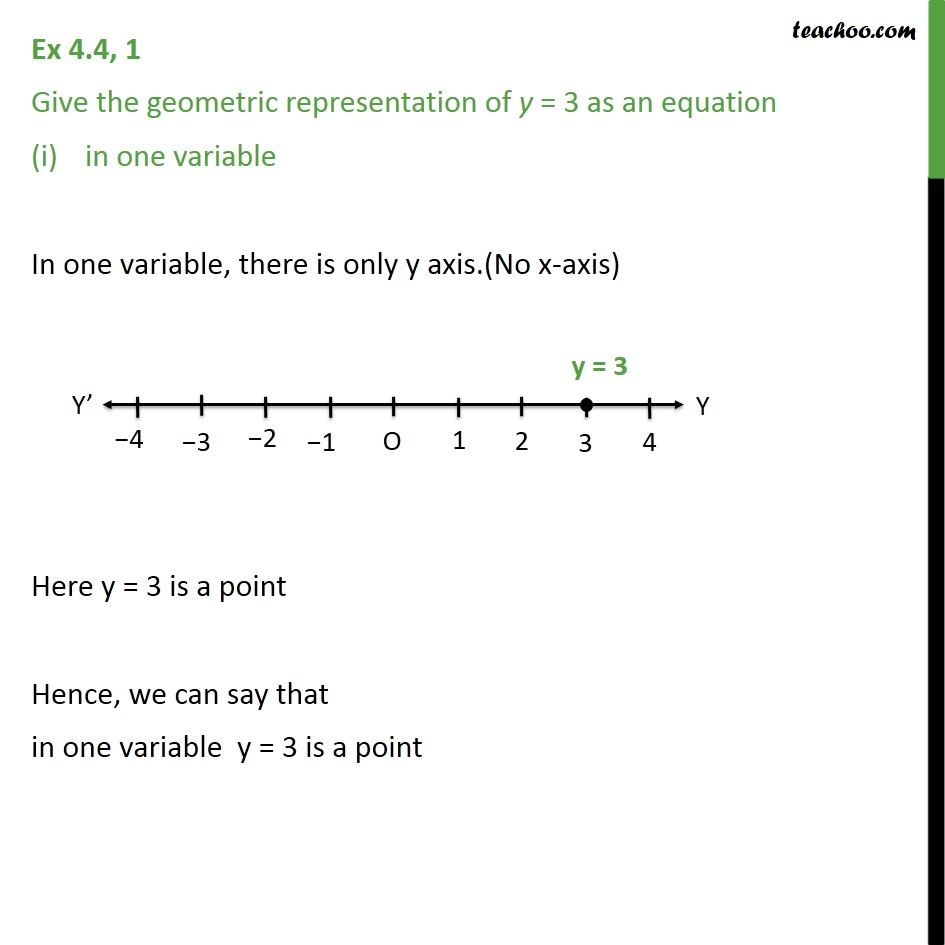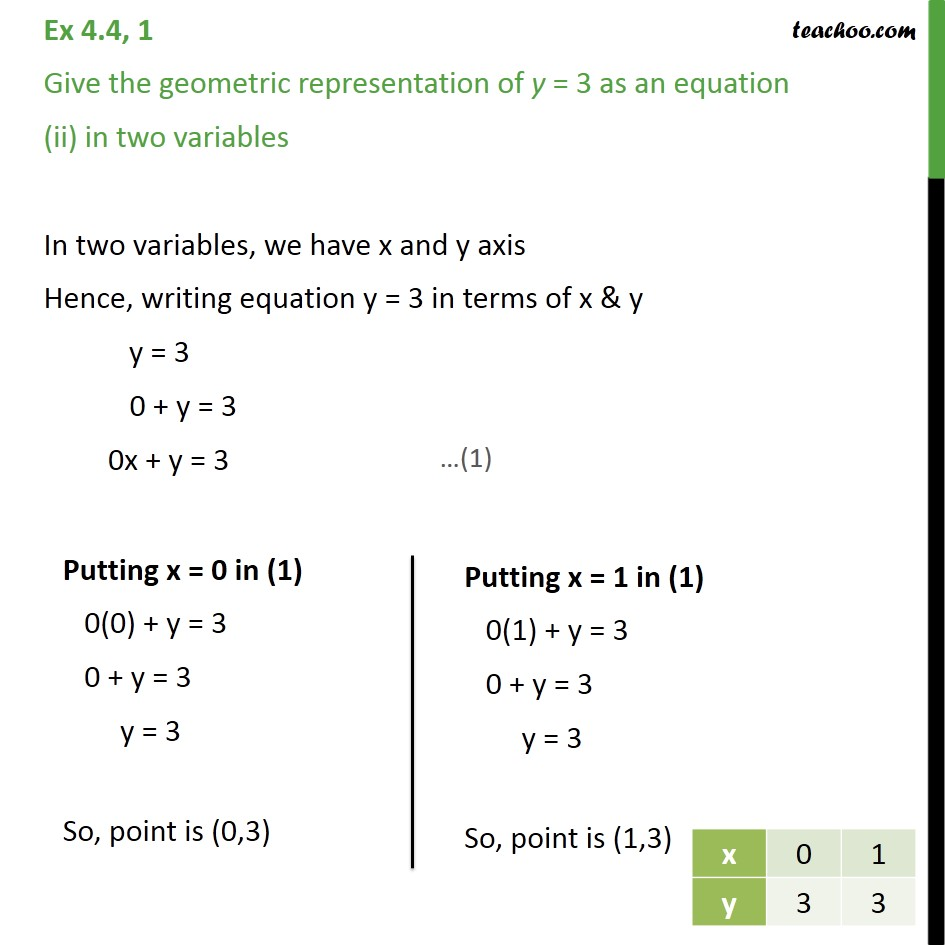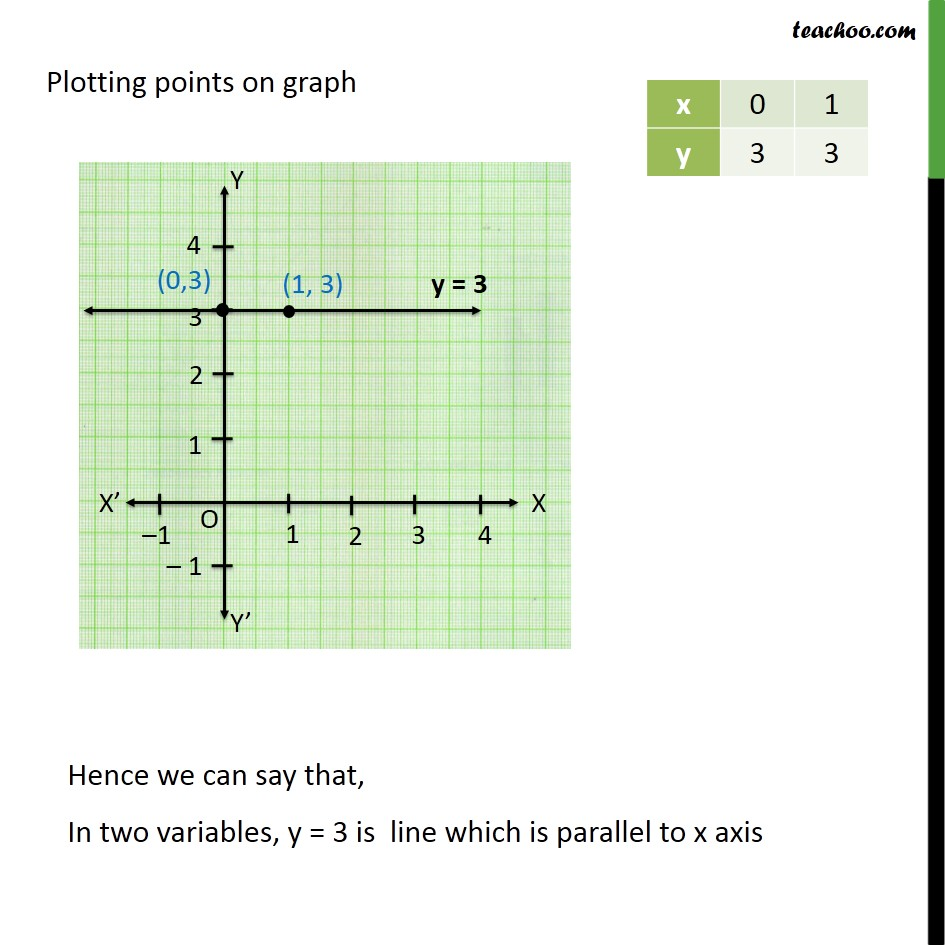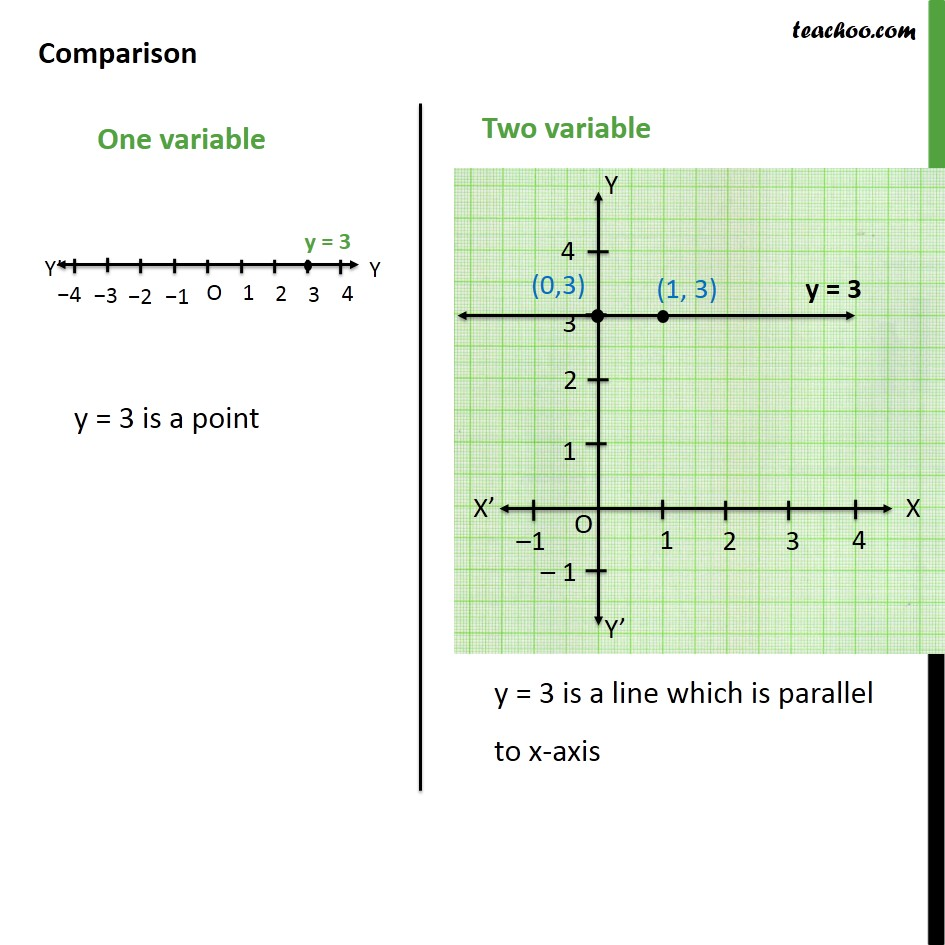Lines parallel x or y axis

Chapter 4 Class 9 Linear Equations in Two Variables
Concept wiseLearn in your speed, with individual attention - Teachoo Maths 1-on-1 Class

### Transcript

Question 1 Give the geometric representation of y = 3 as an equation in one variable In one variable, there is only y axis.(No x-axis) Here y = 3 is a point Hence, we can say that in one variable y = 3 is a point Question 1 Give the geometric representation of y = 3 as an equation (ii) in two variables In two variables, we have x and y axis Hence, writing equation y = 3 in terms of x & y y = 3 0 + y = 3 0x + y = 3 Plotting points on graph Comparison